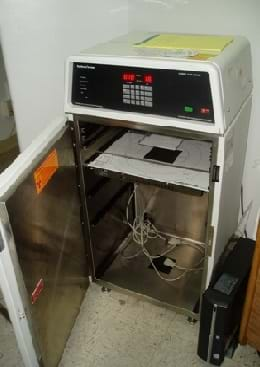# Hands-on ActivityLinear Regression of Bone Mineral Density Scanners

(0 Ratings)

### Quick Look

Grade Level: 11 (9-12)

Time Required: 45 minutes

Expendable Cost/Group: US \$0.00

This activity requires the use of non-expendable (reusable) graphing calculators, one per student.

Group Size: 1

Activity Dependency:

Subject Areas: AlgebraA small specimen bone mineral density scanner.

### Summary

Students complete an exercise showing logarithmic relationships and examine how to find the linear regression of data that does not seem linear upon initial examination. They relate number of BMD scanners to time.

### Engineering Connection

Rarely in the real world does data collected by scientists and engineers fit an exact equation. Finding the equation of the line that fits the data best is a large part of what all engineers do when they collect and analyze data. In this activity, students practice this skill, especially as it involves using natural logarithms.

### Learning Objectives

After this activity, students should be able to:

• Use the linear regression function on a graphing calculator.
• Explain how the natural logarithm can be used to linearize data.

### Educational Standards Each TeachEngineering lesson or activity is correlated to one or more K-12 science, technology, engineering or math (STEM) educational standards. All 100,000+ K-12 STEM standards covered in TeachEngineering are collected, maintained and packaged by the Achievement Standards Network (ASN), a project of D2L (www.achievementstandards.org). In the ASN, standards are hierarchically structured: first by source; e.g., by state; within source by type; e.g., science or mathematics; within type by subtype, then by grade, etc.

###### Common Core State Standards - Math
• Fit a function to the data; use functions fitted to data to solve problems in the context of the data. (Grades 9 - 12) More Details

Do you agree with this alignment?

• (+) Understand the inverse relationship between exponents and logarithms and use this relationship to solve problems involving logarithms and exponents. (Grades 9 - 12) More Details

Do you agree with this alignment?

###### International Technology and Engineering Educators Association - Technology
• Analyze how technology transfer occurs when a user applies an existing innovation developed for one function to a different purpose. (Grades 9 - 12) More Details

Do you agree with this alignment?

###### Tennessee - Math
• (+) Understand the inverse relationship between exponents and logarithms and use this relationship to solve problems involving logarithms and exponents. (Grades 9 - 12) More Details

Do you agree with this alignment?

• Fit a function to the data; use functions fitted to data to solve problems in the context of the data. (Grades 9 - 12) More Details

Do you agree with this alignment?

###### Tennessee - Science
• Scientific Research (Grades 9 - 12) More Details

Do you agree with this alignment?

Suggest an alignment not listed above

### Subscribe

Get the inside scoop on all things TeachEngineering such as new site features, curriculum updates, video releases, and more by signing up for our newsletter!
PS: We do not share personal information or emails with anyone.

### Materials List

Each student needs:

### More Curriculum Like This

High School LessonCommon and Natural Logarithms and Solving Equations

Students continue an examination of logarithms in the Research and Revise stage by studying two types of logarithms—common logarithms and natural logarithm. They take notes about the two special types of logarithms, why they are useful, and how to convert to these forms by using the change of base f...

High School LessonStatistical Analysis of Flexible Circuits

Students are introduced to the technology of flexible circuits, some applications and the photolithography fabrication process. They are challenged to determine if the fabrication process results in a change in the circuit dimensions since, as circuits get smaller and smaller (nano-circuits), this c...

### Pre-Req Knowledge

Students must be comfortable using graphing calculators (though detailed instructions are included). Students must also be familiar with linear graphing.

### Introduction/Motivation

Now that we have learned the basics of natural logarithms, let's do a real-world activity that uses natural logarithms. What you are going to do with the provided data is something that many engineers do all the time. All types of engineers use natural logarithms to analyze data and you will be doing just the very basics of this type of data analysis.

### Procedure

Before the Activity

Make copies of the worksheet (and calculator instructions, if needed).

With the Students

Hand out the worksheets and explain the activity. This worksheet asks students to do a series of exercises with a graphing calculator. If students are familiar enough with graphing, they can do it on their own. If not, provide them with the detailed instructions on how to complete the graphing exercise. Note that one step in the instructions asks students to show their graphs to the teacher to check that they understand how to create a graph before moving to the next step.

### Assessment

Activity Embedded Assessment

Graphing Checkpoint: If students use the graphing calculator instructions, there is a checkpoint where the teacher can see if they understand how to correctly graph.

Post-Activity Assessment

Activity Worksheet: Collect and grade the activity worksheet for completion and accuracy. Review their data, graph, answers and work to gauge their mastery of the subject.

### Activity Scaling

• For lower grades, provide the graphing calculator instructions.
• For upper grades, do not provide the graphing calculator instructions.

© 2013 by Regents of the University of Colorado; original © 2006 Vanderbilt University

### Contributors

Kristyn Shaffer; Megan Johnston

### Supporting Program

VU Bioengineering RET Program, School of Engineering, Vanderbilt University

### Acknowledgements

The contents of this digital library curriculum were developed under National Science Foundation RET grant nos. 0338092 and 0742871. However, these contents do not necessarily represent the policies of the NSF, and you should not assume endorsement by the federal government.